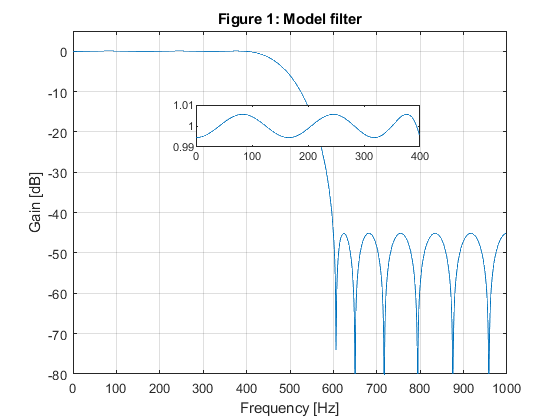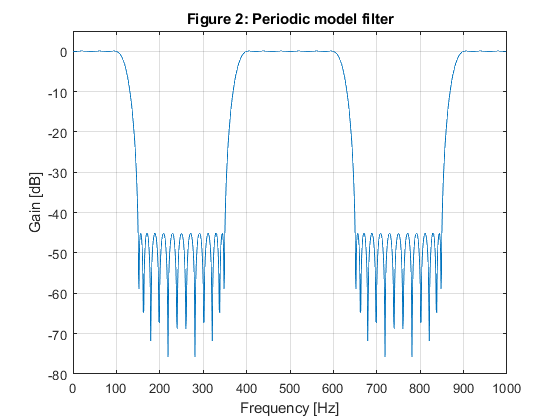# Multirate Filtering for Digital Signal Processing: MATLAB® Applications, Chapter 10: Frequency-Response Masking Techniques - Exercises

## Exercise 10.1

Used function: ifir (Filter Design Toolbox)

Use the MATLAB function ifir to design a lowpass FIR filter to satisfy the specifications of Example 10.1. Compute and plot the magnitude response of the overall lowpass filter and compare the results with that of Example 10.1.

Specifications of Example 10.1 A lowpass FIR filter is designed for Fp = 100 Hz, Fs = 150 Hz, and the sampling frequency of F0 = 2000 Hz. The passband and stopband ripples are delta_p = 0.01, and delta_s = 0.01 (a_s = 40 dB).

close all; clear all;
Fp=100; Fs=150; F0=2000;
delta_p=0.01; delta_s=0.01;
% SOLUTION
[g,h] = ifir(4,'low',[Fp/(F0/2),Fs/(F0/2)],[delta_p,delta_s]);
hIFIR = conv(g,h);
[HIFIR,f] = freqz(hIFIR,1,1000,F0);
figure(1),plot(f,20*log10(abs(HIFIR))),
xlabel('Frequency [Hz]'),ylabel('Gain [dB]');
axis([0,F0/2,-60,5]),grid on;
g(1)=axes('Position',[0.40 0.70 0.40 0.1]);
plot(f(1:100),(abs(HIFIR(1:100)))),axis([0,100,0.99,1.01]);
% Figure 1 displays the gain response of the overall filter, which
% evidently meets the speciffications.
% The plots of Figure 1 are simmilar to those of Figure 10.4 which presents
% the solution of Example 10.1 (from the book).## Exercise 10.2

Design a narrowband filter for the specifications of Example 10.1. The model filter G(z) is an IIR halfband filter, and masking filter F(z) is linear-phase FIR filters. Compute and plot the magnitude responses for G(z), G(zM), F(z), and for the overall filter H(z). Compute the total number of multiplication constants and compare with the solution of Example 10.1.

% Specifications of Example 10.1
% A lowpass FIR filter is designed for Fp = 100 Hz, Fs = 150 Hz, and the sampling frequency of F0 = 2000 Hz.
% The passband and stopband ripples are delta_p = 0.01, and delta_s = 0.01 (a_s = 40 dB).
close all; clear all;
Fp=100; Fs=150; F0=2000;
delta_p=0.01; delta_s=0.01;
% SOLUTION
Ng = 7;                                     % The order of the IIR model filter
[b,a,z,p,k] = halfbandiir(Ng,400/(F0/2));   % Model filter
[G,f] = freqz(b,a,1000,F0);
figure(1),plot(f,20*log10(abs(G))),
xlabel('Frequency [Hz]'),ylabel('Gain [dB]'),
axis([0,F0/2,-80,5]),grid on,
title('Model filter');
g(1)=axes('Position',[0.40 0.70 0.40 0.1]);
plot(f(1:400),(abs(G(1:400)))), axis([0,400,0.99,1.01]);
M = 4;

% Generating the periodic model filter
bm = zeros(size(1:M*length(b)));
bm(1:M:length(bm)) = b;
am = zeros(size(1:M*length(a)));
am(1:M:length(am)) = a;
[Gm,f] = freqz(bm,am,1000,F0);
figure(2),plot(f,20*log10(abs(Gm))),
xlabel('Frequency [Hz]'),ylabel('Gain [dB]'),
axis([0,F0/2,-80,5]),grid on,
title('Periodic model filter');

% Design of the masking filter
f0=[0,100/1000,375/1000,650/1000,850/1000,1]; m0=[1,1,0,0,0,0];
Nf = 19; % Length of FIR linear-phase masking filtr
ff = firpm(Nf-1,f0,m0,[0.5,1,1]);
[F,f]=freqz(ff,1,1000,F0);
figure(3),plot(f,20*log10(abs(F))),
xlabel('Frequency [Hz]'),ylabel('Gain [dB]'),
axis([0,F0/2,-80,5]),grid on,
g(1)=axes('Position',[0.45 0.70 0.40 0.1]);
plot(f(1:100),(abs(F(1:100)))), axis([0,100,0.99,1.01]);

% Narrow-band filter characteristic
H=F.*Gm;
figure(4),plot(f,20*log10(abs(H))),
xlabel('Frequency [Hz]'),ylabel('Gain [dB]'),
axis([0,1000,-80,5]),grid on,
title('Narrow-band filter');
g(1)=axes('Position',[0.40 0.70 0.40 0.1]);
plot(f(1:100),(abs(H(1:100)))), axis([0,100,0.99,1.01]);

% Computing the number of multiplication constants N_MULT:
N_MULT = (Ng-1)/2 + (Nf-1)/2 +1
% The total number of multiplication constants is N_MULT = 13, whereas
% the number of multiplication constants in the solution of Example 10.1
% is 16.

N_MULT =

13## Exercise 10.3

Used function: firhalfband (Filter Design Toolbox)

Using the frequency-response masking approach design a highpass FIR filter to satisfy the following specifications: Fp = 900 Hz, Fs = 850 Hz, and the sampling frequency of F0 = 2000 Hz. The passband and stopband ripples are δp = 0.01, and δs = 0.01 (as = 40 dB). Compute and plot the magnitude responses for the following filters: model filter, periodic model filter, masking filter, and for the resulting highpass filter.

close all; clear all;
% SOLUTION
g = firhalfband(22,400/1000);               % Model filter
[G,f]=freqz(g,1,1000,2000);
figure(1),plot(f,20*log10(abs(G))),
xlabel('Frequency [Hz]'),ylabel('Gain [dB]'),
axis([0,1000,-80,5]),grid on,
title('Model filter');
gg(1)=axes('Position',[0.35 0.65 0.40 0.1]);
plot(f(1:400),(abs(G(1:400)))), axis([0,400,0.99,1.01]);

% Generation of the periodic model filter
gm = zeros(size(1:4*length(g)));
gm(1:4:length(gm)) = g;
[Gm,f]=freqz(gm,1,1000,2000);
figure(2),plot(f,20*log10(abs(Gm))),
xlabel('Frequency [Hz]'),ylabel('Gain [dB]'),
axis([0,1000,-80,5]),grid on,
title('Periodic model filter');

% Design of the highpass masking filter
f0=[0,125/1000,375/1000,625/1000,900/1000,1]; m0=[0,0,0,0,1,1];
ff = firpm(18,f0,m0,[0.5,0.5,1]);
[F,f]=freqz(ff,1,1000,2000);
figure(3),plot(f,20*log10(abs(F))),
xlabel('Frequency [Hz]'),ylabel('Gain [dB]'),
axis([0,1000,-80,5]),grid on,
g(1)=axes('Position',[0.25 0.75 0.40 0.1]);
plot(f(900:1000),(abs(F(900:1000)))), axis([900,1000,0.99,1.01]);

% Narrow-band filter characteristic
H=F.*Gm;
figure(4),plot(f,20*log10(abs(H))),
xlabel('Frequency [Hz]'),ylabel('Gain [dB]'),
axis([0,1000,-80,5]),grid on,
title('Narrow-band filter');
g(1)=axes('Position',[0.25 0.65 0.40 0.1]);
plot(f(900:1000),(abs(H(900:1000)))), axis([900,1000,0.99,1.01]);## Exercise 10.4

Design a wideband linear-phase lowpass filter specified with the passband edge frequency Fp = 1100 Hz, the stopband edge frequency Fs = 1350 Hz and the sampling frequency F0 = 4000 Hz. The maximal ripple in the passband is ap = ±0.1 dB, and minimal stopband attenuation amounts to as = 40 dB. Compute and plot the magnitude responses for the following filters: model filter, periodic model filter, masking filters, and for the resulting lowpass filter.

close all; clear all;
% SOLUTION
% Design od the model filter
omegap = 2*pi*1100/4000;
omegas = 2*pi*1350/4000;
M = 4;                                      % We chose
omegaGs = omegas*M - 2*pi;                  % Model filter stopband angular edge frequency
fGs = omegaGs/pi;                           % Model filter stopband edge frequency
omegaGp = omegap*M - 2*pi;                  % Model filter passband angular edge frequency
fGp = omegaGp/pi;                           % Model filter passpband edge frequency
% Selecting the suitable passband edge frequency for the halfband model filter:
if 1-fGs>=fGp,
fGp = 1-fGs;
end;
%fGp = 1-fGs;                               % Model filter passband edge frequency
g = firhalfband(22,fGp);                    % Model filter design
[G,fr] = freqz(g,1,1000,4000);
figure(1),plot(fr,20*log10(abs(G))),
xlabel('Frequency [Hz]'),ylabel('Gain [dB]'),
axis([0,2000,-100,5]),grid on,
title('Model filter');

% Design of the masking filters F0(z) and F1(z)
ap = 0.08; as = 40;
delta_p = (10^(ap/20)-1)/(10^(ap/20)+1);    % Passband ripple
delta_s = 10^(-as/20);                      % Stopband ripple
fpF0 = (2+fGp)/M; fsF0 = (4-fGs)/M;         % Boundary frequencies for filter F0(z)
freq = [0,fpF0,fsF0,1]; m0 = [1,1,0,0]; w = [delta_s/delta_p,1]; % Seting design parameters for masking filter F0(z)
f0 = firpm(18,freq,m0,w);                   % Designing the masking filter f0
fpF1 = (2-fGp)/M; fsF1 = (2+fGs)/M;         % Boundary frequencies for filter F1(z)
freq = [0,fpF1,fsF1,1]; m1=[1,1,0,0];       % Seting design parameters for masking filter  F1(z
f1 = firpm(20,freq,m1,w);                   % Designing the masking filter f1
[F0,fr] = freqz(f0,1,1000,4000);
[F1,fr] = freqz(f1,1,1000,4000);
figure(2),plot(fr,20*log10(abs(F0)),fr,20*log10(abs(F1)),'--');grid
xlabel('Frequency [Hz]'),ylabel('Gain [dB]'),
axis([0,2000,-60,5]),grid on,
xlabel('Frequency [Hz]'),ylabel('Gain [dB]'),
f0=[0,f0,0];                                % Equalization of the masking filters' delays

% Generation of the periodic model filter
gm = zeros(size(1:4*length(g)));
gm(1:4:length(gm)) = g;                   % Periodic model filter
[Gm,fr] = freqz(gm,1,1024,2);
figure(3),plot(fr,20*log10(abs(Gm)));
xlabel('\omega/\pi'),ylabel('Gain [dB]'),
axis([0,1,-100,5]),grid on,
title('Periodic model filter');

% Implementation of the wide-band filter according to Figure 10.8
d = zeros(size(gm)); d(45) = 1;             % Delay branch
gmc = d - gm;                               % Complementary periodic model filter
[Gmc,fr]=freqz(gmc,1,1000,4000);
h = conv(gm,f0) + conv(gmc,f1);             % Impulse response of the overall filter
[H,Fr]=freqz(h,1,1000,4000);
figure(4),plot(Fr,20*log10(abs(H))),
xlabel('Frequency [Hz]'),ylabel('Gain [dB]')
hold on
plot([0,1350,1350,2000],[0,0,-40,-40],'r--'),
axis([0,2000,-60,5]), grid on,
title('Wide-band filter');
g(1)=axes('Position',[0.20 0.55 0.40 0.07]);
plot(Fr(1:550),20*log10(abs(H(1:550)))),axis([0,1100,-0.1,0.1]);